#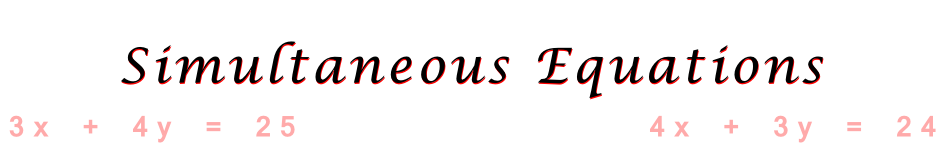There are 366 different Starters of The Day, many to choose from. You will find below some starters on the topic of Simultaneous Equations. A lesson starter does not have to be on the same topic as the main part of the lesson or the topic of the previous lesson. It is often very useful to revise or explore other concepts by using a starter based on a totally different area of Mathematics.

Main Page

### Simultaneous Equations Starters: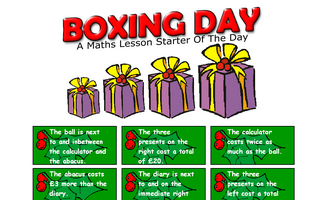Work out the contents and the cost of the Christmas boxes from the given clues.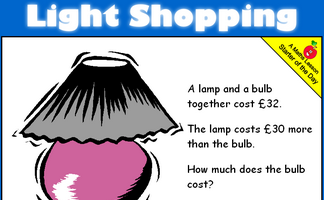A lamp and a bulb together cost 32 pounds. The lamp costs 30 pounds more than the bulb. How much does the bulb cost?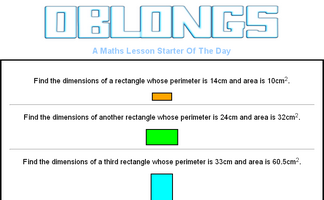Find the dimensions of a rectangle given the perimeter and area.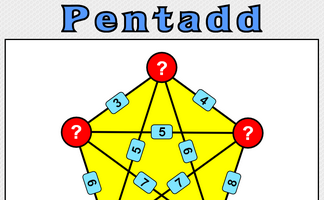Five numbers are added together in pairs and the sums shown. What might the five numbers be?The sum and product are given, can you find the two numbers?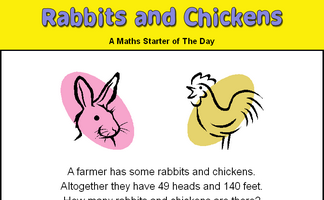There are some rabbits and chickens in a field. Calculate how many of each given the number of heads and feet.Use the weights of the trains to work out the weight of a locomotive and a coach. A real situation which produces simultaneous equations.It is called Refreshing Revision because every time you refresh the page you get different revision questions.A problem involving two people's ages which can be solved using algebra.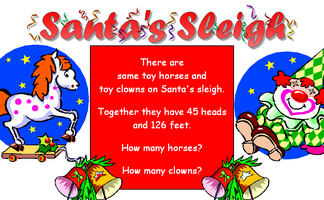Work out the number of clowns and horses given the number of heads and feet.A problem which can best be solved as a pair of simultaneous equations.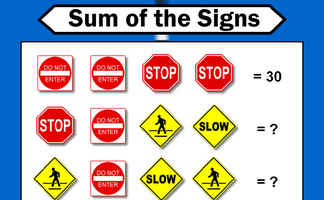Each traffic sign stands for a number. Some of the sums of rows and columns are shown. What numbers might the signs stand for?How many children and how many donkeys are on the beach? You can work it out from the number of heads and the number of feet!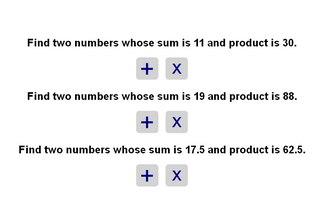Find the two numbers whose sum and product are given.

## Exercises#### Algebra In Action

Real life problems adapted from an old Mathematics textbook which can be solved using algebra.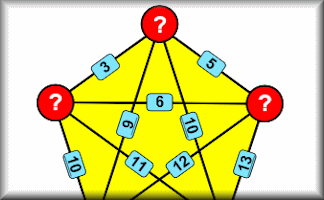Find the five numbers which when added or multiplied together in pairs to produce the given sums or products.#### Recurring Decimals

Change recurring decimals into their corresponding fractions and vica versa.#### Simultaneous Equations

A self-marking, multi-level set of exercises on solving pairs of simultaneous equations.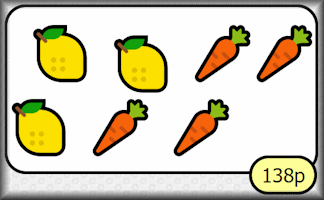#### Simultaneous Shopping

Find the cost of the individual items in shopping baskets (introduction to simultaneous equations).#### Simultaneous Solutions

Arrange the given pairs of simultaneous equations in groups to show whether they have no solution, one solution or infinite solutions.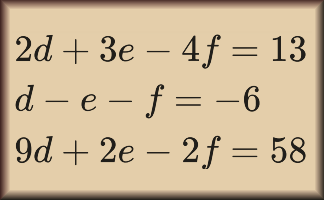#### Three Unknowns

Solve these sets of three simultaneous, linear equations to find the values of the variables.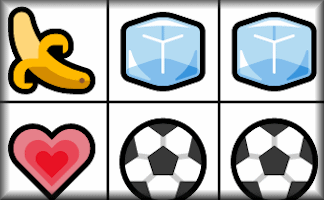#### Unbeknownst

Some picture grid puzzles which can be solved by using simultaneous equations.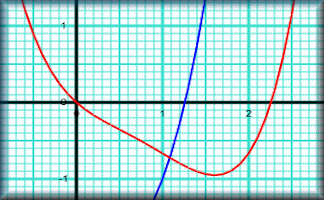#### Using Graphs

Use the graphs provided and create your own to solve both simultaneous and quadratic equations.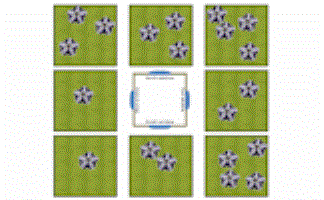#### Window View

Drag the 20 flowers into the gardens so that 9 flowers are visible from each window of the house.

### Search

The activity you are looking for may have been classified in a different way from the way you were expecting. You can search the whole of Transum Maths by using the box below.

Have today's Starter of the Day as your default homepage. Copy the URL below then select
Tools > Internet Options (Internet Explorer) then paste the URL into the homepage field.

Set as your homepage (if you are using Internet Explorer)

Do you have any comments? It is always useful to receive feedback and helps make this free resource even more useful for those learning Mathematics anywhere in the world. Click here to enter your comments.For All: